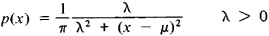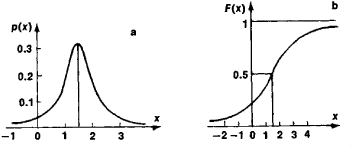# Cauchy Distribution

(redirected from Cauchy noise)

## Cauchy distribution

[kō·shē dis·trə′byü·shən]
(statistics)
A distribution function having the form M /[π M 2+ (x-a)2], where x is the variable and M and a are constants. Also known as Cauchy frequency distribution.
McGraw-Hill Dictionary of Scientific & Technical Terms, 6E, Copyright © 2003 by The McGraw-Hill Companies, Inc.
The following article is from The Great Soviet Encyclopedia (1979). It might be outdated or ideologically biased.

## Cauchy Distribution

a special type of probability distribution of random variables. Introduced by Cauchy, it is marked by the densityThe characteristic function is

f(t) = exp (μit − λ ǀ t ǀ)

The Cauchy distribution is unimodal and symmetric with respect to the point x = μ, which is its mode and median. NoFigure 1. Cauchy distribution: (a) probability density, (b) distribution function

moments of positive order of a Cauchy distribution exist. Figure 1 depicts a Cauchy distribution for μ = 1.5 and λ = 1.

The Great Soviet Encyclopedia, 3rd Edition (1970-1979). © 2010 The Gale Group, Inc. All rights reserved.
Site: Follow: Share:
Open / Close